7 Work and Kinetic Energy

7.2 Kinetic Energy

Learning Objectives

By the end of this section, you will be able to:

• Calculate the kinetic energy of a particle given its mass and its velocity or momentum
• Evaluate the kinetic energy of a body, relative to different frames of reference

It’s plausible to suppose that the greater the velocity of a body, the greater effect it could have on other bodies. This does not depend on the direction of the velocity, only its magnitude. At the end of the seventeenth century, a quantity was introduced into mechanics to explain collisions between two perfectly elastic bodies, in which one body makes a head-on collision with an identical body at rest. The first body stops, and the second body moves off with the initial velocity of the first body. (If you have ever played billiards or croquet, or seen a model of Newton’s Cradle, you have observed this type of collision.) The idea behind this quantity was related to the forces acting on a body and was referred to as “the energy of motion.” Later on, during the eighteenth century, the name kinetic energy was given to energy of motion.

With this history in mind, we can now state the classical definition of kinetic energy. Note that when we say “classical,” we mean non-relativistic, that is, at speeds much less that the speed of light. At speeds comparable to the speed of light, the special theory of relativity requires a different expression for the kinetic energy of a particle, as discussed in Relativity in the third volume of this text.

Since objects (or systems) of interest vary in complexity, we first define the kinetic energy of a particle with mass m.

Kinetic Energy

The kinetic energy of a particle is one-half the product of the particle’s mass m and the square of its speed v: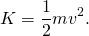We then extend this definition to any system of particles by adding up the kinetic energies of all the constituent particles: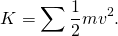Note that just as we can express Newton’s second law in terms of either the rate of change of momentum or mass times the rate of change of velocity, so the kinetic energy of a particle can be expressed in terms of its mass and momentum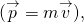instead of its mass and velocity. Since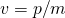, we see that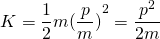also expresses the kinetic energy of a single particle. Sometimes, this expression is more convenient to use than (Figure).

The units of kinetic energy are mass times the square of speed, or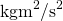. But the units of force are mass times acceleration,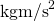, so the units of kinetic energy are also the units of force times distance, which are the units of work, or joules. You will see in the next section that work and kinetic energy have the same units, because they are different forms of the same, more general, physical property.

Example

Kinetic Energy of an Object

(a) What is the kinetic energy of an 80-kg athlete, running at 10 m/s? (b) The Chicxulub crater in Yucatan, one of the largest existing impact craters on Earth, is thought to have been created by an asteroid, traveling at

22 km/s and releasing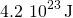of kinetic energy upon impact. What was its mass? (c) In nuclear reactors, thermal neutrons, traveling at about 2.2 km/s, play an important role. What is the kinetic energy of such a particle?

Strategy

To answer these questions, you can use the definition of kinetic energy in (Figure). You also have to look up the mass of a neutron.

Solution

Don’t forget to convert km into m to do these calculations, although, to save space, we omitted showing these conversions.

1.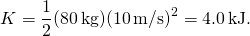2.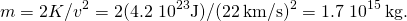3.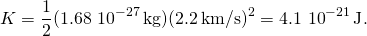Significance

In this example, we used the way mass and speed are related to kinetic energy, and we encountered a very wide range of values for the kinetic energies. Different units are commonly used for such very large and very small values. The energy of the impactor in part (b) can be compared to the explosive yield of TNT and nuclear explosions,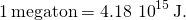The Chicxulub asteroid’s kinetic energy was about a hundred million megatons. At the other extreme, the energy of subatomic particle is expressed in electron-volts,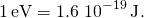The thermal neutron in part (c) has a kinetic energy of about one fortieth of an electron-volt.

(a) A car and a truck are each moving with the same kinetic energy. Assume that the truck has more mass than the car. Which has the greater speed? (b) A car and a truck are each moving with the same speed. Which has the greater kinetic energy?

Because velocity is a relative quantity, you can see that the value of kinetic energy must depend on your frame of reference. You can generally choose a frame of reference that is suited to the purpose of your analysis and that simplifies your calculations. One such frame of reference is the one in which the observations of the system are made (likely an external frame). Another choice is a frame that is attached to, or moves with, the system (likely an internal frame). The equations for relative motion, discussed in Motion in Two and Three Dimensions, provide a link to calculating the kinetic energy of an object with respect to different frames of reference.

Example

Kinetic Energy Relative to Different Frames

A 75.0-kg person walks down the central aisle of a subway car at a speed of 1.50 m/s relative to the car, whereas the train is moving at 15.0 m/s relative to the tracks. (a) What is the person’s kinetic energy relative to the car? (b) What is the person’s kinetic energy relative to the tracks? (c) What is the person’s kinetic energy relative to a frame moving with the person?

Strategy

Since speeds are given, we can use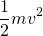to calculate the person’s kinetic energy. However, in part (a), the person’s speed is relative to the subway car (as given); in part (b), it is relative to the tracks; and in part (c), it is zero. If we denote the car frame by C, the track frame by T, and the person by P, the relative velocities in part (b) are related by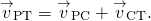We can assume that the central aisle and the tracks lie along the same line, but the direction the person is walking relative to the car isn’t specified, so we will give an answer for each possibility,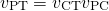, as shown in (Figure).Figure 7.10 The possible motions of a person walking in a train are (a) toward the front of the car and (b) toward the back of the car.

Solution

1.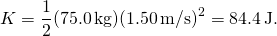2.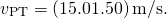Therefore, the two possible values for kinetic energy relative to the car are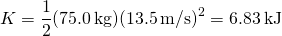and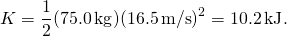3. In a frame where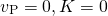as well.

Significance

You can see that the kinetic energy of an object can have very different values, depending on the frame of reference. However, the kinetic energy of an object can never be negative, since it is the product of the mass and the square of the speed, both of which are always positive or zero.

You are rowing a boat parallel to the banks of a river. Your kinetic energy relative to the banks is less than your kinetic energy relative to the water. Are you rowing with or against the current?

The kinetic energy of a particle is a single quantity, but the kinetic energy of a system of particles can sometimes be divided into various types, depending on the system and its motion. For example, if all the particles in a system have the same velocity, the system is undergoing translational motion and has translational kinetic energy. If an object is rotating, it could have rotational kinetic energy, or if it’s vibrating, it could have vibrational kinetic energy. The kinetic energy of a system, relative to an internal frame of reference, may be called internal kinetic energy. The kinetic energy associated with random molecular motion may be called thermal energy. These names will be used in later chapters of the book, when appropriate. Regardless of the name, every kind of kinetic energy is the same physical quantity, representing energy associated with motion.

Example

Special Names for Kinetic Energy

(a) A player lobs a mid-court pass with a 624-g basketball, which covers 15 m in 2 s. What is the basketball’s horizontal translational kinetic energy while in flight? (b) An average molecule of air, in the basketball in part (a), has a mass of 29 u, and an average speed of 500 m/s, relative to the basketball. There are about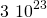molecules inside it, moving in random directions, when the ball is properly inflated. What is the average translational kinetic energy of the random motion of all the molecules inside, relative to the basketball? (c) How fast would the basketball have to travel relative to the court, as in part (a), so as to have a kinetic energy equal to the amount in part (b)?

Strategy

In part (a), first find the horizontal speed of the basketball and then use the definition of kinetic energy in terms of mass and speed,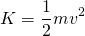. Then in part (b), convert unified units to kilograms and then useto get the average translational kinetic energy of one molecule, relative to the basketball. Then multiply by the number of molecules to get the total result. Finally, in part (c), we can substitute the amount of kinetic energy in part (b), and the mass of the basketball in part (a), into the definition, and solve for v.

Solution

1. The horizontal speed is (15 m)/(2 s), so the horizontal kinetic energy of the basketball is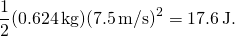2. The average translational kinetic energy of a molecule is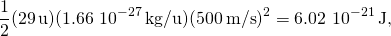and the total kinetic energy of all the molecules is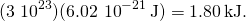3.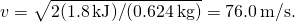Significance

In part (a), this kind of kinetic energy can be called the horizontal kinetic energy of an object (the basketball), relative to its surroundings (the court). If the basketball were spinning, all parts of it would have not just the average speed, but it would also have rotational kinetic energy. Part (b) reminds us that this kind of kinetic energy can be called internal or thermal kinetic energy. Notice that this energy is about a hundred times the energy in part (a). How to make use of thermal energy will be the subject of the chapters on thermodynamics. In part (c), since the energy in part (b) is about 100 times that in part (a), the speed should be about 10 times as big, which it is (76 compared to 7.5 m/s).

Summary

• The kinetic energy of a particle is the product of one-half its mass and the square of its speed, for non-relativistic speeds.
• The kinetic energy of a system is the sum of the kinetic energies of all the particles in the system.
• Kinetic energy is relative to a frame of reference, is always positive, and is sometimes given special names for different types of motion.

Conceptual Questions

A particle of m has a velocity of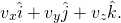Is its kinetic energy given by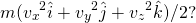If not, what is the correct expression?

One particle has mass m and a second particle has mass 2m. The second particle is moving with speed v and the first with speed 2v. How do their kinetic energies compare?

The first particle has a kinetic energy of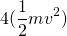whereas the second particle has a kinetic energy of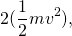so the first particle has twice the kinetic energy of the second particle.

A person drops a pebble of mass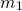from a height h, and it hits the floor with kinetic energy K. The person drops another pebble of mass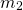from a height of 2h, and it hits the floor with the same kinetic energy K. How do the masses of the pebbles compare?

Problems

Compare the kinetic energy of a 20,000-kg truck moving at 110 km/h with that of an 80.0-kg astronaut in orbit moving at 27,500 km/h.

(a) How fast must a 3000-kg elephant move to have the same kinetic energy as a 65.0-kg sprinter running at 10.0 m/s? (b) Discuss how the larger energies needed for the movement of larger animals would relate to metabolic rates.

a. 1.47 m/s; b. answers may vary

Estimate the kinetic energy of a 90,000-ton aircraft carrier moving at a speed of at 30 knots. You will need to look up the definition of a nautical mile to use in converting the unit for speed, where 1 knot equals 1 nautical mile per hour.

Calculate the kinetic energies of (a) a 2000.0-kg automobile moving at 100.0 km/h; (b) an 80.-kg runner sprinting at 10. m/s; and (c) a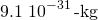electron moving at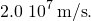a. 772 kJ; b. 4.0 kJ; c.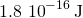A 5.0-kg body has three times the kinetic energy of an 8.0-kg body. Calculate the ratio of the speeds of these bodies.

An 8.0-g bullet has a speed of 800 m/s. (a) What is its kinetic energy? (b) What is its kinetic energy if the speed is halved?

a. 2.6 kJ; b. 640 J